# 手把手教你用Python构建你的第一个多标签图像分

https://www.analyticsvidhya.com/blog/2019/01/build-image-classification-model-10-minutes/?utm_source=blog&utm_medium=multi-label-image-classification 可思数据-www.sykv.cn,sykv.com

可思数据1. 什么是多标签图像分类?

2. 多标签图像分类与多类图像分类有何不同?

3. 了解多标签图像分类模型体系结构; 可思数据-AI,智能驾驶,人脸识别,区块链,大数据

4. 构建多标签图像分类模型的步骤;

5. 案例研究：用Python解决多标签图像分类问题;

6.接下来的步骤和你的实验;

7.尾记。 内容来自可思数据

1. 什么是多标签图像分类?可思数据-AI,智能驾驶,人脸识别,区块链,大数据可思数据

2. 多标签图像分类与多类图像分类有何不同?

可思数据-数据挖掘,智慧医疗,机器视觉,机器人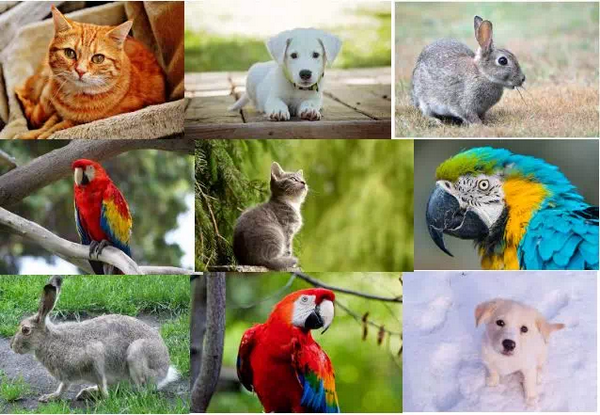可思数据-www.sykv.cn,sykv.com可思数据-AI,人工智能,深度学习,机器学习,神经网络

https://www.analyticsvidhya.com/blog/2019/01/build-image-classification-model-10-minutes/?utm_source=blog&utm_medium=multi-label-image-classification

3. 构建多标签图像分类模型的步骤 本文来自可思数据，转载请联系本站及注明出处

4. 了解多标签图像分类模型结构

5. 案例研究：用Python解决多标签图像分类问题

可思数据可思数据-AI,人工智能,深度学习,机器学习,神经网络

1. import keras 可思数据-数据挖掘,智慧医疗,机器视觉,机器人

2. from keras.models import Sequential

3. from keras.layers import Dense, Dropout, Flatten 可思数据-数据挖掘,智慧医疗,机器视觉,机器人

4. from keras.layers import Conv2D, MaxPooling2D

5. from keras.utils import to_categorical 可思数据-数据挖掘,智慧医疗,机器视觉,机器人

6. from keras.preprocessing import image 可思数据-数据挖掘,智慧医疗,机器视觉,机器人

7. import numpy as np 可思数据

8. import pandas as pd

9. import matplotlib.pyplot as plt 可思数据-www.sykv.cn,sykv.com

10. from sklearn.model_selection import train_test_split 本文来自可思数据，转载请联系本站及注明出处

11. from tqdm import tqdm

12. %matplotlib inline 本文来自可思数据，转载请联系本站及注明出处

1. train = pd.read_csv('multi_label_train.csv') # reading the csv file 可思数据-数据挖掘,智慧医疗,机器视觉,机器人

2. train.head() # printing first five rows of the file 可思数据-数据挖掘,智慧医疗,机器视觉,机器人

可思数据-www.sykv.cn,sykv.com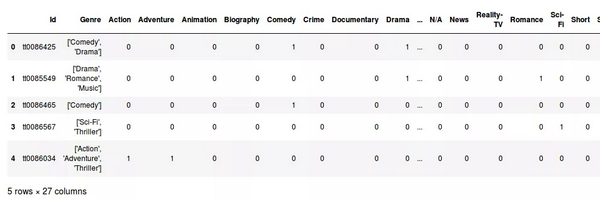1. train.columns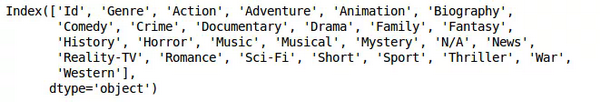可思数据-AI,智能驾驶,人脸识别,区块链,大数据

Genre列包含每个图像的列表，其中明确了每个图像对应的电影的类型。因此，从.csv文件的头部开始，第一个图像的类型是喜剧和戏剧。 本文来自可思数据，转载请联系本站及注明出处

1. train_image = [] 内容来自可思数据

2. for i in tqdm(range(train.shape)): 可思数据

3. img = image.load_img('Multi_Label_dataset/Images/'+train['Id'][i]+'.jpg',target_size=(400,400,3)) 可思数据-数据挖掘,智慧医疗,机器视觉,机器人

4. img = image.img_to_array(img) 可思数据-数据挖掘,智慧医疗,机器视觉,机器人

5. img = img/255 可思数据-www.sykv.cn,sykv.com

6. train_image.append(img) 可思数据-人工智能资讯平台

7. X = np.array(train_image)

1. X.shape 可思数据-www.sykv.cn,sykv.com

本文来自可思数据，转载请联系本站及注明出处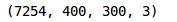1. plt.imshow(X)内容来自可思数据

1. train['Genre']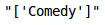可思数据-www.sykv.cn,sykv.com

1. y = np.array(train.drop(['Id', 'Genre'],axis=1))
2. y.shape可思数据-数据挖掘,智慧医疗,机器视觉,机器人

1. X_train, X_test, y_train, y_test = 可思数据-人工智能资讯平台

2. train_test_split(X, y, random_state=42, test_size=0.1)

1. model = Sequential()

2. model.add(Conv2D(filters=16, kernel_size=(5, 5), activation="relu", input_shape=(400,400,3)))

3. model.add(MaxPooling2D(pool_size=(2, 2)))

4. model.add(Dropout(0.25)) 可思数据-数据挖掘,智慧医疗,机器视觉,机器人

5. model.add(Conv2D(filters=32, kernel_size=(5, 5), activation='relu'))

6. model.add(MaxPooling2D(pool_size=(2, 2))) 本文来自可思数据，转载请联系本站及注明出处

7. model.add(Dropout(0.25))

8. model.add(Conv2D(filters=64, kernel_size=(5, 5), activation="relu")) 可思数据-数据挖掘,智慧医疗,机器视觉,机器人

9. model.add(MaxPooling2D(pool_size=(2, 2)))

10. model.add(Dropout(0.25)) 可思数据-AI,智能驾驶,人脸识别,区块链,大数据

11. model.add(Conv2D(filters=64, kernel_size=(5, 5), activation='relu'))

12. model.add(MaxPooling2D(pool_size=(2, 2))) 可思数据-人工智能资讯平台

13. model.add(Dropout(0.25))

14. model.add(Flatten()) 可思数据-www.sykv.cn,sykv.com

15. model.add(Dense(128, activation='relu'))

16. model.add(Dropout(0.5)) 可思数据

17. model.add(Dense(64, activation='relu')) 可思数据-数据挖掘,智慧医疗,机器视觉,机器人

18. model.add(Dropout(0.5)) 可思数据-www.sykv.cn,sykv.com

19. model.add(Dense(25, activation='sigmoid')) 可思数据-www.sykv.cn,sykv.com

1. model.summary() 可思数据-AI,人工智能,深度学习,机器学习,神经网络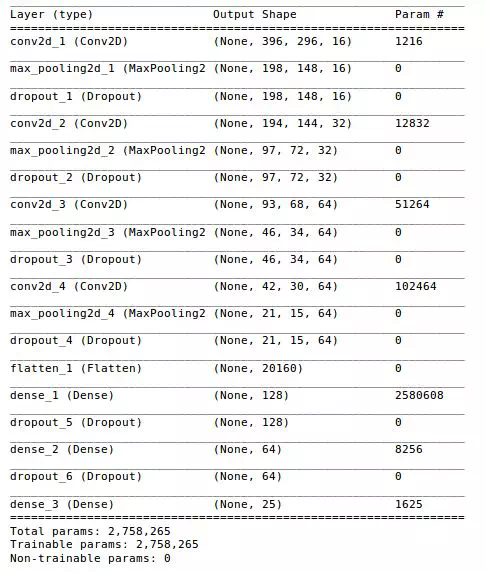1. model.compile(optimizer='adam', loss='binary_crossentropy', metrics=['accuracy'])

1. model.fit(X_train, y_train, epochs=10, validation_data=(X_test, y_test), batch_size=64)

可思数据-数据挖掘,智慧医疗,机器视觉,机器人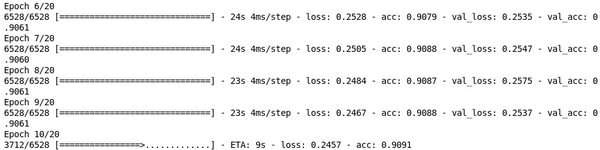内容来自可思数据

GOT 可思数据-人工智能资讯平台

https://drive.google.com/file/d/1cfIE-42H4_UM-JERoctseLUpKwmd40YE/view 可思数据-AI,人工智能,深度学习,机器学习,神经网络

Avengers 可思数据-AI,智能驾驶,人脸识别,区块链,大数据

https://drive.google.com/file/d/1buNOcfo0Im2HmFH778dUwxven8Zzebtu/view

1. img = image.load_img('GOT.jpg',target_size=(400,400,3))

2. img = image.img_to_array(img) 可思数据-AI,人工智能,深度学习,机器学习,神经网络

3. img = img/255

1. classes = np.array(train.columns[2:]) 本文来自可思数据，转载请联系本站及注明出处

2. proba = model.predict(img.reshape(1,400,400,3))

3. top_3 = np.argsort(proba)[:-4:-1]

4. for i in range(3):

5. print("{}".format(classes[top_3[i]])+" ({:.3})".format(proba[top_3[i]]))

6. plt.imshow(img)

本文来自可思数据，转载请联系本站及注明出处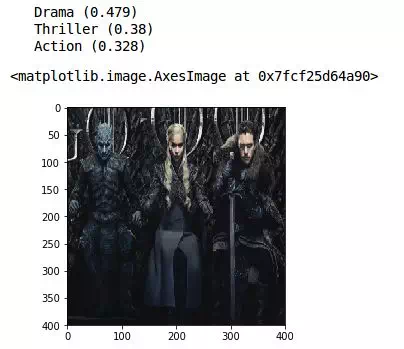可思数据-www.sykv.cn,sykv.com

1. img = image.load_img('avengers.jpeg',target_size=(400,400,3)) 可思数据-AI,人工智能,深度学习,机器学习,神经网络

2. img = image.img_to_array(img) 可思数据-www.sykv.cn,sykv.com

3. img = img/255

1. classes = np.array(train.columns[2:]) 内容来自可思数据

2. proba = model.predict(img.reshape(1,400,400,3))

3. top_3 = np.argsort(proba)[:-4:-1] 可思数据-数据挖掘,智慧医疗,机器视觉,机器人

4. for i in range(3): 可思数据-人工智能资讯平台

5. print("{}".format(classes[top_3[i]])+" ({:.3})".format(proba[top_3[i]]))

6. plt.imshow(img)

可思数据-www.sykv.cn,sykv.com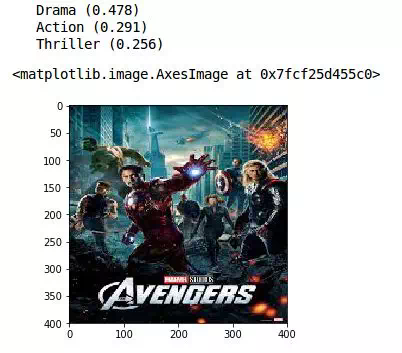可思数据-AI,智能驾驶,人脸识别,区块链,大数据1. img = image.load_img('golmal.jpeg',target_size=(400,400,3))

2. img = image.img_to_array(img) 可思数据-人工智能资讯平台

3. img = img/255

1. classes = np.array(train.columns[2:])

2. proba = model.predict(img.reshape(1,400,400,3)) 可思数据-数据挖掘,智慧医疗,机器视觉,机器人

3. top_3 = np.argsort(proba)[:-4:-1]

4. for i in range(3): 本文来自可思数据，转载请联系本站及注明出处

5. print("{}".format(classes[top_3[i]])+" ({:.3})".format(proba[top_3[i]])) 可思数据-AI,人工智能,深度学习,机器学习,神经网络

6. plt.imshow(img) 可思数据-数据挖掘,智慧医疗,机器视觉,机器人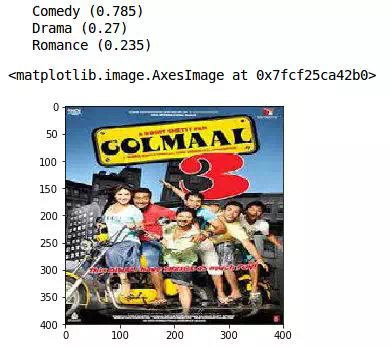可思数据-www.sykv.cn,sykv.com

《Golmaal 3》是一部喜剧，我们的模型预测它为最受欢迎的类型。其他预测类型是剧情片和浪漫片——相对准确的评估。我们可以看到该模型能够仅通过海报预测电影类型。

6. 接下来的步骤和你自己的实验

7. 尾记

https://www.analyticsvidhya.com/blog/2019/04/build-first-multi-label-image-classification-model-python/ 可思数据

Build your First Multi-Label Image Classification Model in Python

Copyright©2005-2019 Sykv.com 可思数据 版权所有    网站地图   联系我们